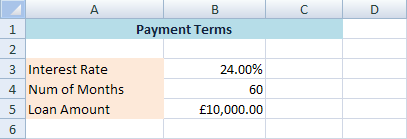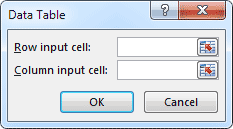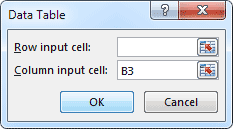# Excel Data Tables

In Excel, a Data Table is a way to see different results by altering an input cell in your formula. As an example, we're going to alter the interest rate, and see how much a £10,000 loan would cost each month. The interest rate will be our input cell. By asking Excel to alter this input, we can quickly see the different monthly payments. Want to know how much we'd pay back each month if the interest was 24 percent per year. But other banks may be offering better deals. So we'll ask Excel to calculate how much we'd pay each month if the interest rate was 22 percent a year, 20 percent a year, and 18 percent a year.

The formula we need is the Payment one you met in a previous section - PMT( ). Here it is again:

PMT(rate, nper, pv, fv, type)

We only need the first three arguments. So for us, it's just this:

PMT(rate, nper, pv)

Rate means the interest rate. The second argument, nper, is how many months you've got to pay the loan back. The third argument, pv, is how much you want to borrow.

Let's make a start then. On a new spreadsheet, set up the following labels:So we'll put our starting interest rate in cell B3 (rate), our loan length in cell B4 (nper), and our loan amount in cell B5 (pv).

Enter the following in cells B3 to B5:So you need to enter 24.00% in cell B3, 60 in cell B4, and £10,000 in cell B5.

We'll enter our formula now. Click inside cell D2 and enter the following:

=PMT(B3 / 12, B4, -B5)

Cell B3 is the interest rate. But this is for the entire year. In the formula, we're diving whatever is in cell B3 by 12. This will get us a monthly interest rate. B4 in the formula is the number of months, which is 60 for us. B5 has a minus sign before it. It's a minus figure because it's a debt.

When you press the enter key on your keyboard, Excel should give you an answer of £287.68.

Now that we have our function in place, we can create an Excel Data Table. First, though, we need to tell Excel about those other interest rates. It will use these to work out the new monthly payments. Remember, Excel is recalculating the PMT function. So it needs some new values to calculate with.

So enter some new values in cells C3, C4, and C5. Enter the same ones as in the image below:We have put the PMT function in cell D2 for a reason. This is one Row up, and one Column to the right of our first new interest rate of 22%. The new monthly payments are going to go in cells D3 to D5. Excel needs the table setting out this way.

So that Excel can work out the new totals, you have to highlight both the new values and the Function you're using.

So highlight the cells C2 to D5. Your spreadsheet should look like this:As you can see, the cells C2 to D5 are now highlighted. This includes our new interest rate values in the C column, and our PMT function in cell D2. We can now create an Excel Data Table. This will work out new monthly payemnts for us. So do this:

• From the Excel menu bar, click on Data
• Locate the Data Tools panel
• Click on the "What if Analysis" item:In Excel 2016, the What If Analysis is on the Forecast panel, to the right of Data Tools:When you click on the "What if Analysis" item, you'll see the following menu:Click on Data Table, and you'll see this small dialogue box:In the dialogue box, there is only a Row input cell or a Column input cell. We want Excel to fill downwards, down a column. So we need the second text box on the dialogue box "Column input cell". If we were filling across in rows, we would use the "Row input cell" text box.

The Input Cell for us is the one that contains our original interest rate. This is the cell you want Excel to substitute.

So click inside the Column input cell box and enter B3:Click OK. When you do, Excel will work out the new monthly payments:So if we could get an 18 percent interest rate, our monthly payments would be £253.93.

If you click inside any of the cells D3 to D5, then look at the formula bar, you will see this:

{=TABLE(,B3)}

That's Excel's way of telling you that a Table has been created, based on the input cell B3

We'll try one more Data Table in the next part. We'll try an easier formula, this time

<--Back to the Excel Contents Page